ICSE Class 9 Maths Sample Question Paper 7 with Answers

Section – A [40-Marks]
(Attempt all questions from this Section)

Question 1.
(a) If, in a ∆ABC, AB = 3 cm, BC = 4 cm and ∠ABC = 90°, find the values of cos C, sin C and
tan C.
Given : AB = 3 cm, BC = 4 cm, ∠ABC = 90°
By Pythagoras theorem,
AC2 = AB2 + BC2 = 32 + 42 = 25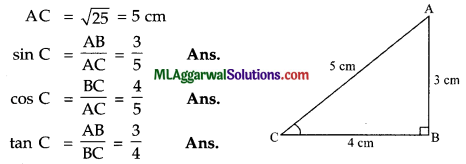(b) A man purchased an old scooter for ₹ 16,000. If the cost of the scooter after 2 years depreciates to ₹ 14,440, find the rate of depreciation.
Present value (V0) = ₹ 16,000
Value after 2 year (V1) = ₹ 14,440
∴ n =2
Let r be the rate of depreciation.(c) Prove that √2 + √5 is irrational.
Let us assume that √2 + √5 is a rational number.
Then $$\sqrt{2}+\sqrt{5}=\frac{a}{b}$$
Where a and b co-prime positive integers.
$$\frac{a}{b}-\sqrt{2}=\sqrt{5}$$Question 2.
(a) If $$x=\frac{1}{x-2 \sqrt{3}}$$ , find the values of (i) x – $$\frac{1}{x}$$ (ii) x + $$\frac{1}{x}$$.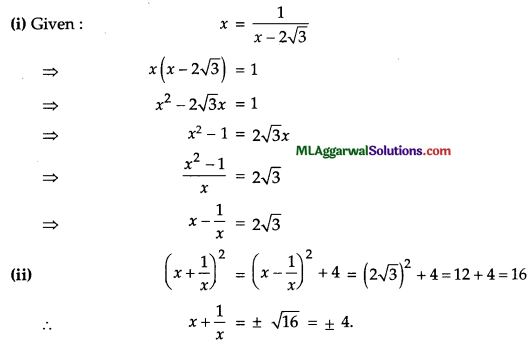(b) In the given figure, ABC is an equilateral triangle. Find the measures of angles marked by x, y and z.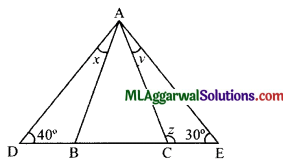Given : ABC is an equilateral triangle.
∠ABC = ∠ACB = ∠B AC = 60°.
Now, ∠BAD + ∠ADB = ∠ABC (Ext. angle is equal to sum of int. opp. angles)
⇒ x + 40° = 60°
⇒ x = 60° – 40°
⇒ x = 20°.
Also, ∠CAE + ∠AEC = ∠ACB (Ext. angle is equal to sum of int. opp. angles)
⇒ y + 30° = 60°
⇒ y = 60° – 30°
⇒ y = 30°
and ∠ACE +∠ACB = 180° (Linear Pair)
⇒ z + 60° = 180°
⇒ z = 180° – 60°
⇒ z = 120°(c) Solve $$\frac{2}{3} x^{2}-\frac{1}{3} x-1=0$$
$$\frac{2}{3} x^{2}-\frac{1}{3} x-1=0$$
$$3 \times \frac{2}{3} x^{2}-3 \times \frac{1}{3} x-3 \times 1=3 \times 0$$
⇒ 2x2 – x – 3 = 0
⇒ 2 x 2 – (3 – 2)x -3=0
⇒ 2x2 – 3x + 2x – 3 = 0
⇒ x (2x – 3) + 1 (2x – 3) = 0
⇒ (2x – 3) (x + 1) = 0
⇒ 2x-3=0 or x + 1= 0
⇒ x= $$\frac{3}{2}$$ or x =-1
⇒ x= $$\frac{3}{2}$$ or -1

Question 3.
(a) Factorize : a3 – b3 – a + b.
a3 -b3 – a + b = (a-b) (a2 + ab + b2) – (a-b) = (a-b) (a2 + ab + b2 – 1).

(b) Draw a histogram to represent the following :

 Class Interval 40 – 48 48-56 56-64 64-72 72 – 80 Frequency 15 25 35 30 10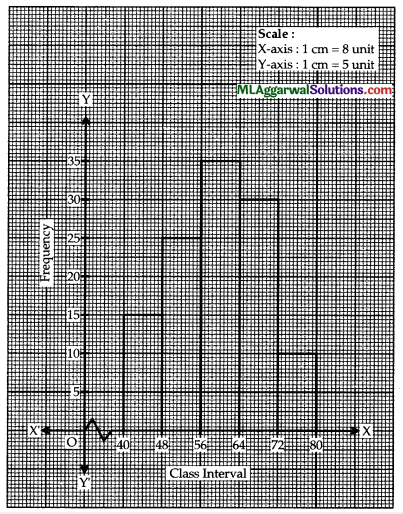(c) Prove that $$\sqrt{\frac{1-\sin 30^{\circ}}{1+\sin 30^{\circ}}}=\tan 30^{\circ}$$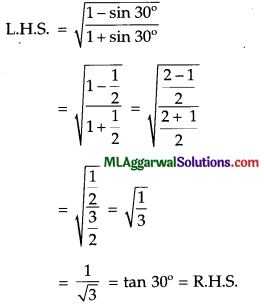Question 4.
(a) Simplify: $$\frac{5^{2(x+6)} \times(25)^{-7+2 x}}{(125)^{2 x}}$$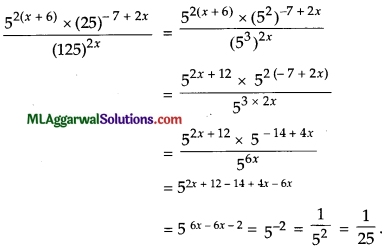(b) In the figure, DE||BC. Prove that (i) Area of ΔACD = Area of ΔABE (ii) Area of ΔOBD = Area of ΔOCE.Given DE || BC
Area of ΔBCD = Area of ΔBCE
(Triangles on same base and between same parallels have equal area) Now, Area of ΔACD + Area of ΔBCD = Area of ΔABE + Area of ΔBCE
⇒ Area of ΔACD = Area of ΔABE (∵ Area of ABCD = Area of ABCE).
Hence Proved.

(ii) Area of ABCD = Area of ABCE [From (i)]
⇒ Area of ABCD – Area of ΔOBC = Area of ΔBCE – Area of ΔOBC
(Subtracting area of ΔOBC from both side)
⇒ Area of ΔOBD = Area of ΔOCE.
Hence Proved

(c) If log10 x + $$\frac{1}{3}$$ log10 y = 1, express y in terms of x.
Given log10 x + $$\frac{1}{3}$$ log10y = 1
log10 x + log10 y1/3 = log10 10
log10 (xy1/3) = log10 10Section – B
(Attempt any four questions from this Section)

Question 5.
(a) The mean of 9 observations was found to be 35. Later on, it was detected that an observation 81 was misread as 18. Find the correct mean of the observations.
We know,
Σx =$$\bar{x}$$ x n
Incorrect ∑ x = 35 x 9 = 315
Correct ∑ x =315 – 18 + 81 = 378
Correct mean = $$\frac{378}{9}=42$$

(b) In the given figure, O is the centre of the circle. AB and CD are two chords of the circle. OM is perpendicular to AB and ON is perpendicular to CD. AB = 24 cm, OM = 5 cm, ON = 12 cm. Find (i) radius of the circle (ii) Length of chord CD.⇒ 169 =144 ÷ CN2
⇒ CN2 = (169 – 144) = 25
⇒ CN= √25 =5
⇒ CD =2 CN (∵ N is mid-point of CD)
⇒ 2 x 5 = 10cm.(c) If $$x^{2}+\frac{1}{x^{2}}=83$$ find the value of $$x^{3}-\frac{1}{x^{3}}$$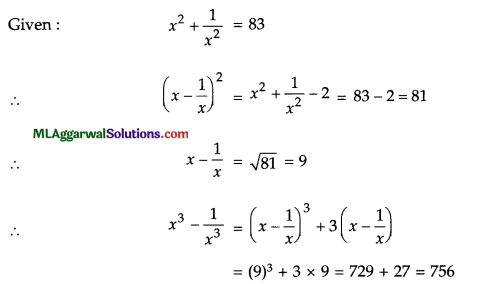Question 6.
(a) A cumulative frequency distribution is given below. Convert this into a frequency distribution table.

 Marks Below 45 Below 60 Below 75 Below 90 Below 105 Below 120 No. of Students 0 8 23 48 85 116

 Marks No. of Students Class Interval Frequency Below 45 0 0-45 0 Below 60 8 45 – 60 8 (8-0) Below 75 23 60 – 75 15 (23 – 8) Below 90 48 75 – 90 25 (48 – 23) Below 105 85 90 -105 37 (85 – 48) Below 120 116 105 – 120 31 (116 – 85)(b) Half the perimeter of a garden, whose length is 4 more than its width, is 36 m. Find the dimensions of the garden.
Let length and breadth of the garden be x m and y m respectively.
According to the question,
x = 4 + y …(i)
and x + y = 36 …(ii)
Substituting x = 4 + y in equation (ii), we get
4 + y + y = 36
2y = 36 – 4
y = $$\frac{32}{2}$$ = 16
Substituting y= 16 in equation (i), we get
x = 4 + 16 = 20
∴ Length = 20 m and breadth = 16 m.

(c) If x and y are rational numbers and $$\frac{5+2 \sqrt{3}}{7+4 \sqrt{3}}=x-y \sqrt{3}$$Question 7.
(a) Factorize : (x2 + y2 – z2)2 – 4x2y2.
(x2 + y2 – z2) – 4x2y2 = (x2 + y1 – z2)2 – (2xy)2
= (x2 + y2 – z2 + 2xy) (x2 + y2 – z2 – 2xy)
= {(x2 + y2 + 2xy) – z2} {(x2 + y2 – 2xy) – z2}
= {(x + y)2 – (z)2}  – y)2 – (z)2}
= {x + y + z) {x + y – z) {x – y + z) {x – y – z).(b) Prove that in a right angled triangle, the median drawn to the hypotenuse is half the hypotenuse in length.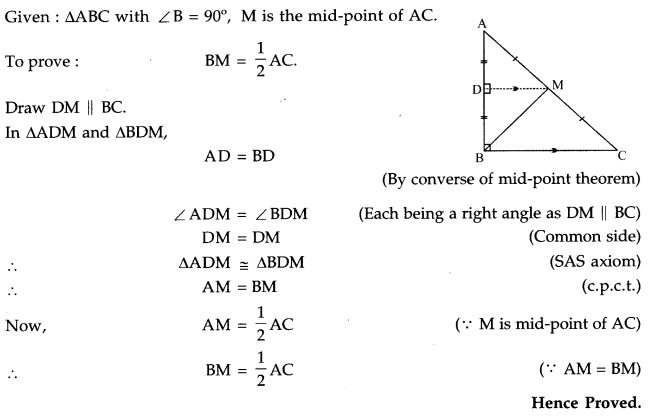(c) Find the value of x if 3 cot2 (x – 5°) = 1.
3 cot2 (x – 5°) =1
1 cot2 (x – 5°) = $$\frac{1}{3}$$
cot (x – 5°) = $$\frac{1}{\sqrt{3}}$$
cot (x – 5°) = cot 60°
x – 5°= 60°
x = 60° + 5°
x = 65°

Question 8.
(a) Solve: $$\frac{x+y}{x y}=2 ; \frac{x-y}{x y}=1$$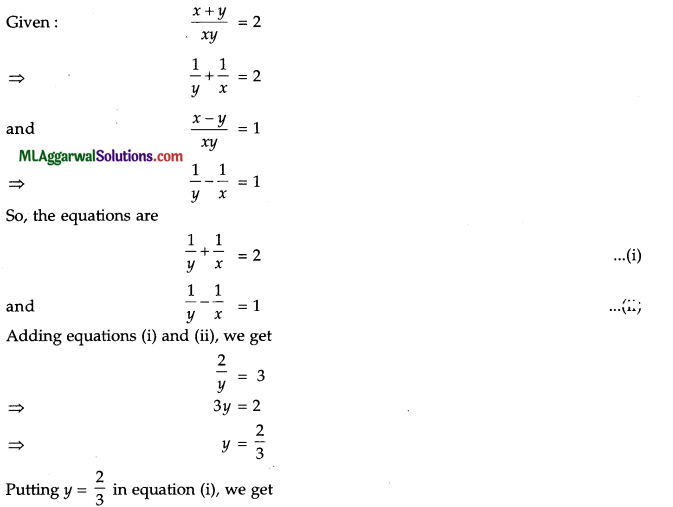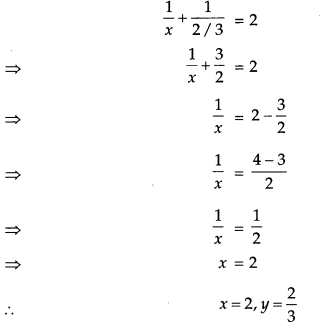(b) Construct a parallelogram ABCD with AB = 5.1 cm, BC = 7 cm and ∠ABC = 75°.
Given: AB =5.1cm, BC = 7cm and ∠ABC = 75°
Steps of construction:
(1) Draw BC=7cm.
(2) At B, draw ∠ XBC = 75°
(3) From B, cut-off BA = 5.1 cm on BX.
(4) From C, draw an arc of radius 5.1 cm.
(5) From A, draw an arc of 7 cm to cut the arc from C at D.
Hence, ABCD is the required parallelogram.(c) Calculate the distance between A (7, 3) and B on the X-axis whose abscissa is 11.
Given : A (7, 3)
∵ B lies on the X-axis whose abscissa is 11, the coordinates of B are (11, 0)
$$\mathrm{AB}=\sqrt{(11-7)^{2}+(0-3)^{2}}=\sqrt{4^{2}+(-3)^{2}}=\sqrt{16+9}=\sqrt{25}$$
= 5 Units.Question 9.
(a) A sum of money ₹ 15,000 amounts to ₹ 16,537.50 in x years at the rate of 5% p.a. compounded annually. Find x.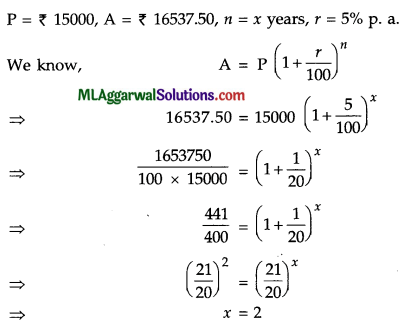(b) In the given figure, ∠PSR = 90°, PQ = 10 cm, QS = 6 cm and RQ = 9 cm. Calculate the length of PR.Given: ∠PSR = 90°, PQ = 10 cm, QS = 6 cm, RQ = 9 cm.
∴ In Δ PQ2, PQ2 = PS2 + QS2 (Pythagoras theorem)
102 =PS2+62
PS2 = 100 – 36
PS = √64 = 8cm
In ΔPRS, PR2 = PS2 + RS2 (Pythagoras theorem)
PR2 = 8 + (9 + 6)2 = 64 + 225 = 289
PR=√289=17cm.

(c) In the given figure, ACB is a semicircle whose radius is 10.5 cm and C is a point on the semicircle at a distance of 7 cm from B. Find the area of the shaded region.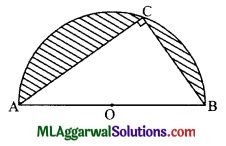For semi-circle,
r = 10.5 cm
∴ Area =$$\text { Area }=\frac{1}{2} \pi r^{2}=\frac{1}{2} \times \frac{22}{7} \times(10.5)^{2}=173.25 \mathrm{~cm}^{2}$$
For triangle ABC,
AB2 = BC2 + AC2 (Pythagoras theorem, ∠C = 90°)
(2 x 10.5)2 = 72 ÷ AC2
AC2 =441 – 49 =392
AC = 19.8 cm.
Area = x BC x AC = x 7 x 19.8 = 69.3 cm2
The area of shaded region = (173.25 – 69.3) cm2
= 103.95 cm2Question 10.
(a) If a2 + b2 + c2 – ab – be – ca = 0, prove that a = b = c.
Given a2 + b2 + c2 – ab – be – ca =0
⇒ 2 (a2 + b2 + c2 – ab – be – ca) = 0
⇒  2a2 + 1b2 + 2c2 -2ab – 2bc – 2ca = 0
⇒ (a2 – 2ab + b2) + (b2 – 2be + c2) + (c2 – 2ca + a2) = 0
⇒ (a – b)2 + (b – c)2 + (c – a)2 =0
The above expression is possible only if
⇒ (a- b)2 = 0 Ab- c)2 = 0, (c – a)2 = 0
a-b =0, b – c = 0, c-a = 0
a = b,b = c, c = a
a = b = c.
Hence Prove.

(b) Solve graphically x + 3y = 6; 2x – 3y = 12 and hence find the value of a, if Ax + 3y = a
x+3y=6 ………. (i)
2x – 3y = 12 ….. (ii)
from (i)  x = 6 – 3y

 X 6 3 0 y 0 1 2

∴ (6, 0), (3, 1), (0, 2)
From (ii),
2x = 3y + 12
x = $$\frac{3 y+12}{2}$$

 X 6 3 0 y 0 1 2

(6, 0), (3, – 2), (0, – 4)
These points are piotted in the graph.The two lines intersect at the point (6, 0).
∴ x = 6, y = 0
Now 4x + 3 y = a
⇒ 4 x 6 + 3 x 0 = a
24 + 0 =a
⇒ a = 24(c) Given, 1008 = 2p.3q.7r, find the values of p, q, r and hence evaluate 2p.3q.7-r÷192.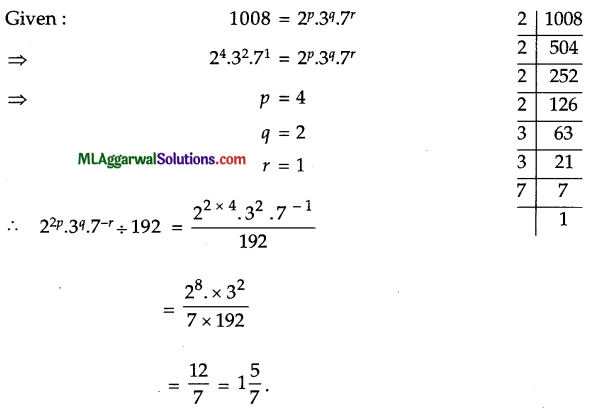Question 11.
(a) If log $$\frac{x-y}{2}=\frac{1}{2}$$(log x + log y), prove that x2 + y2 = 6xy.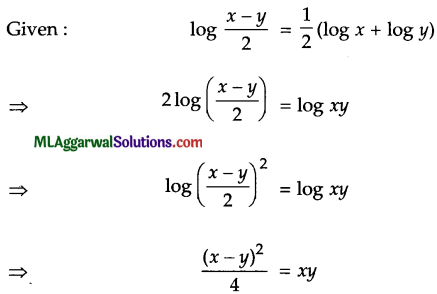x2 + y2 – 2xy = 4xy
x2 + y2 – 4xy + 2 xy
x2 + y2 = 6 xy.
Hence Proved

(b) In a pentagon ABCDE, AB||ED and ∠B = 140°. Find ∠C and ∠D if ∠C: ∠D = 5:6.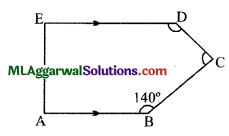Given : AB||ED, ZB = 140°, ∠C : ∠D = 5:6.
Let  ∠C =5x, ∠D = 6x.
Now,∠A+∠E= 180° (Co-interior angles, AB||ED)
Also, ∠A+ ∠B+ ∠C+ ∠D+ ∠E= (5-2) x 180°
(∠A + ∠E) + ∠B + ∠C + ∠D
= 3 x 180° 180° + 140° + 5x + 6x = 540°
11 x = 540° – 320°
$$x=\frac{220^{\circ}}{11}$$
∠C = 5x = 5 x 20° = 100°
∠D = 6x = 6 x 20° = 120°(c) Factorize : 4 a3b – 44 a2b + 112